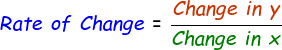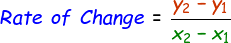# Rate of Change Calculator

The rate of change is the term that is used for measuring the change in y quantity in relation to x quantity. That is,Which can be written as,Here is a sample rate of change graph which shows exactly where the co-ordinates lie and can be considered while calculating rate of change.Use our below online rate of change calculator by entering the difference in y coordinates and x coordinates and then click calculate to find the output.

 x1: x2: y1: y2: Rate of Change:

Latest Calculator Release

Average Acceleration Calculator

Average acceleration is the object's change in speed for a specific given time period. ...

Free Fall Calculator

When an object falls into the ground due to planet's own gravitational force is known a...

Torque Calculator

Torque is nothing but a rotational force. In other words, the amount of force applied t...

Average Force Calculator

Average force can be explained as the amount of force exerted by the body moving at giv...

Angular Displacement Calculator

Angular displacement is the angle at which an object moves on a circular path. It is de...